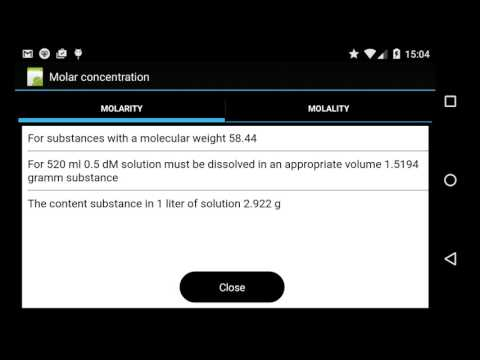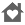# Molarity and molality calculatorEveryoneThis free calculator allows you to calculate how much to weigh the material to obtain a solution of desired molarity or molality. Also you can calculate molar mass of substances. App contain Periodic table of elements. The help file provides basic concepts and examples.
Via application knowing the molar mass of solute and the molarity of the solution of the substance can be calculated the amount of solute.
The application can be useful to researchers, students and teachers, especially those specializing in molecular biology, biochemistry, microbiology, genetics, chemistry, etc.
The application can read the results of calculations aloud with text-to-speech engine.
1. In the field "Molar concentration" write the molecular weight of the solute. For example, for NaCl mass of the sodium atom is 22.99, the mass of an atom of chlorine - 35,453, a total molecular weight is about 22.99 + 35.45 = 58.44
The masses of the atoms of simple substances can be found in the periodic table, it is called with the "?" or count in the application (click the "Table" button on the right side of the screen)
2. In the field "Molecular weight of the solute" write the desired molarity. If you need any nanomolar or micromolar solution, the application can use the field selection metric prefixes.
3. In the field "Volume of the mixture, ml" write how much we need a solution.
4. Press "Enter".
5. The application calculates the required weight of the substance.
6. Weigh substance.
7. Solute in a volume of the solvent, a little less than the required amount of solution. When dissolution is complete, bring the volume up to the desired value.

In addition, you can calculate the molality of the solution in the application.
Molality, also called molal concentration, is a measure of the concentration of a solute in a solution in terms of amount of substance in a specified amount of mass of the solvent. This contrasts with the definition of molarity which is based on a specified volume of solution (see Wikipedia)

You can calculate the molar mass inside of application
For example, we need to calculate the molar mass of slaked lime Ca(OH)2
Press icon with table.
Press the key with the first letter of the chemical symbol of calcium - in this case, "C". The program will form a list of all the chemical elements, the symbol of which begins at "C" (for example, it will include carbon, cadmium, etc.)

From the list choose calcium. The program displays it at the top of the screen, and displays various
characteristics of calcium. In the center of the screen appears the inscription "Molar mass XXX g/mol", the molar mass

You can edit the molecular weight of the element, for example, change it for uranium from tabular 238.0289 to 235.0439 - molecular weight of a particular isotope. The change will be during the current session.

We repeat the procedure until we introduce the entire formula of the substance.

You can find help here
http://www.homebear.ru/molar/help_en.php
http://colorblind.space/polit_win.txt
Collapse

Review Policy

## What's New

Bug in Android 7+ fixed
Collapse

Eligible for Family LibraryLearn More
Updated
October 21, 2018
Size
2.5M
Installs
10+
Current Version
Version 1.24
Requires Android
4.0.3 and up
Content Rating
Everyone
Permissions
Offered By
denik
Developer
344090, г. Ростов-на-Дону, пр.Стачки 184/1